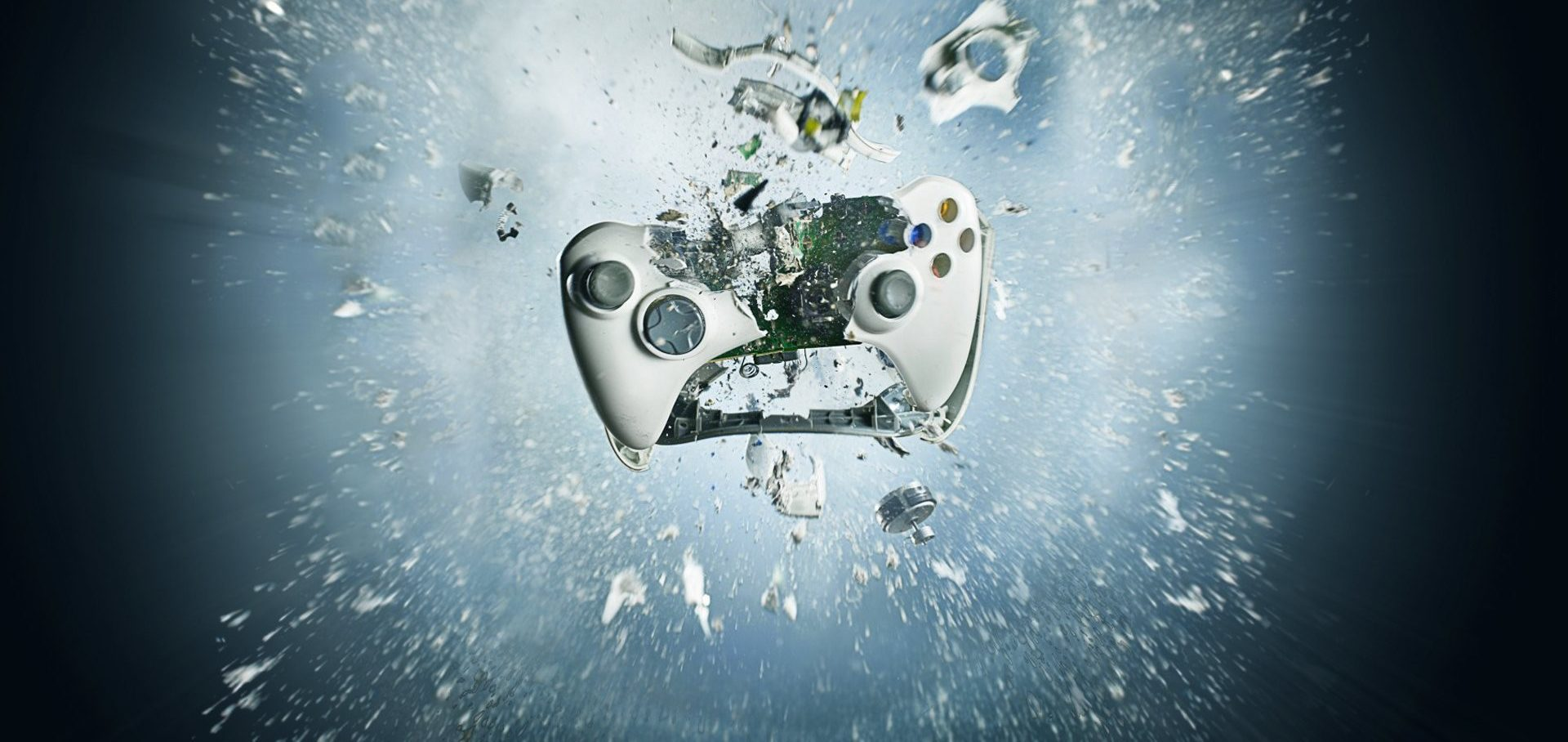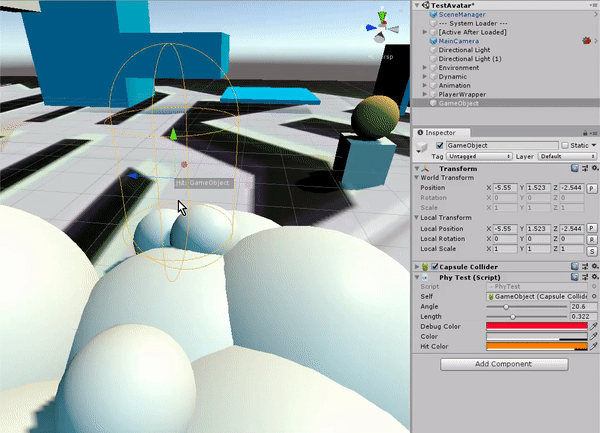# 筆記: 自定義物理 Physics.ComputePenetration

We always want to redefine physics during game development.
usually implement it by tweening or custom path to complete ignore the physic engine result.
however at some point we still wanted to respect the result from physic engine.

Ref : https://docs.unity3d.com/ScriptReference/Physics.ComputePenetration.html

in Unity3D, there is an API : Physics.ComputePentration to handle this situation.
Ref : https://docs.unity3d.com/ScriptReference/Physics.ComputePenetration.html

This function is useful to write custom depenetration functions. One particular example is an implementation of a character controller where a specific reaction to collision with the surrounding physics objects is required. In this case, one would first query for the colliders nearby using OverlapSphere and then adjust the character’s position using the data returned by ComputePenetration.

As you can see based on the capsule position changed, the result from ComputePenetration are always pointing to the avoid collision direction.
that’s what physic engine will do on your object to avoid those collide overlap each others.
however you need to handle each collision vector by yourself, it return `direction` and `distance` and only able to return one object,
so for multiple objects you will need to calculate the final direction & distance after combine all of it.

Here is the implement of above test script.

```using UnityEngine;
using Kit;

[RequireComponent(typeof(CapsuleCollider))]
public class PhyTest : MonoBehaviour
{
[SerializeField] CapsuleCollider m_Self = null;
[SerializeField, Range(0f, 89f)] float m_Angle = 3f;
[SerializeField, Range(0f, 1f)] float m_Length = .3f;
[SerializeField] Color m_DebugColor = Color.yellow;

[SerializeField] Color m_Color = new Color(1f, 1f, 1f, 0.3f);
[SerializeField] Color m_HitColor = new Color(1f, 0f, 0f, 0.4f);
private Collider[] m_OverlapColliders = new Collider;
private int HitCount { get; set; } = 0;

private Vector3 SuggestedDirectionFromEngine(Collider[] objArr, int validRange)
{
Vector3 finalVector = Vector3.zero;
float distance = 0f;
int total = 0;
for (int i = 0; i < validRange; i++)
{
Collider obstacle = objArr[i];
if (obstacle == m_Self)
continue;
Vector3 pushBackDirection;
float pushBackDistance;
bool isCollision = Physics.ComputePenetration(m_Self, transform.position, transform.rotation,
obstacle, obstacle.transform.position, obstacle.transform.rotation, out pushBackDirection, out pushBackDistance);
if (!isCollision)
continue;

total++;
distance += pushBackDistance;
finalVector += pushBackDirection;

Debug.DrawRay(transform.position, pushBackDirection * pushBackDistance, Color.magenta, 0f, false);
}

return finalVector.normalized * (distance / (float)total);
}

private void Reset()
{
if (m_Self == null)
m_Self = GetComponent<CapsuleCollider>();
}

private void OnDrawGizmos()
{
if (KitBox.IsEditorMode)
{
Matrix4x4 matrix = Matrix4x4.TRS(transform.position, transform.rotation, transform.lossyScale);
float half = Mathf.Clamp((m_Self.height / 2f) - m_Self.radius, 0f, float.PositiveInfinity);
Vector3 p0 = matrix.MultiplyPoint3x4(m_Self.center + new Vector3(0f, half, 0f));
Vector3 p1 = matrix.MultiplyPoint3x4(m_Self.center + new Vector3(0f, -half, 0f));
HitCount = Physics.OverlapCapsuleNonAlloc(p0, p1, m_Self.radius, m_OverlapColliders);
}

Vector3 dir = SuggestedDirectionFromEngine(m_OverlapColliders, HitCount);
GizmosExtend.DrawRay(transform.position, dir, Color.green);

GizmosExtend.DrawArrow(transform.position, dir, m_DebugColor, m_Angle, m_Length);
}
}```